# U Substitution and Changing the Limits of Integration

Katherine Kaylegian-Starkey, Carmen Andert, Christianlly Cena
• Author
Katherine Kaylegian-Starkey

Katherine has a bachelor's degree in physics, and she is pursuing a master's degree in applied physics. She currently teaches struggling STEM students at Lane Community College.

• Instructor
Carmen Andert

Carmen has two master's degrees in mathematics has has taught mathematics classes at the college level for the past 9 years.

• Expert Contributor
Christianlly Cena

Christianlly has taught college Physics, Natural science, Earth science, and facilitated laboratory courses. He has a master's degree in Physics and is currently pursuing his doctorate degree.

Understand how to use the U substitution method to calculate definite and indefinite integrals. Learn how to incorporate a change of the limits of integration. Updated: 01/25/2022

Show

## Change of Variables Integration

Sometimes, in calculus, complex integrands can look impossible to solve, and some integrals are only evaluated with computers. For other integrals, noticing patterns within the integrand can make it easier to solve using the appropriate methods. One such method involves changing the variables of integration through a process called U-substitution, where u is a generic variable that replaces the variable of integration, x. U-substitution skips the derivative chain rule, and if an integrand involves a composite function in the form {eq}f(g(x))g'(x) {/eq}. U-substitution is the best way to make the integral solvable. Figure 1 gives the integrands that have trigonometric substitutions.An error occurred trying to load this video.

Try refreshing the page, or contact customer support.

Coming up next: Using Integration By Parts

### You're on a roll. Keep up the good work!

Replay
Your next lesson will play in 10 seconds
• 0:04 Expressing the Same Idea
• 0:38 U-Substitution
• 1:38 Example One
• 3:48 Example Two
• 5:07 Trigonometric Substitution
• 8:16 Lesson Summary
Save Save

Want to watch this again later?

Timeline
Autoplay
Autoplay
Speed Speed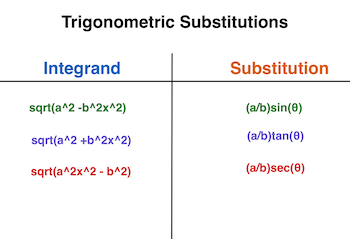## U-Substitution to Find the Definite Integral

A definite integral, written as {eq}\int_a^b f(x) dx {/eq}, is an integral with boundaries, where f(x) is some function, a is the lower boundary of the integral, and b is the upper boundary of the integral. When calculating a U-substitution definite integral, because the variable of integration changes, it is crucial also to change the boundaries of the integral. The basic method for using U-substitution to perform definite integral substitution and appropriately change the bounds of the integral follows these steps:

1) Properly identify that the integral is written as {eq}\int_a^b f(g(x))g'(x) dx {/eq}.

2) Set {eq}u = g(x) {/eq} so that {eq}du = g'(x) dx {/eq}.

3) Put u and du in place of g(x) and g'(x)dx so that {eq}\int_a^b f(g(x))g'(x) dx {/eq} becomes {eq}\int_a^b f(u)du {/eq}.

4) The boundaries of integration imply that a and b should be plugged in for x to evaluate the final answer. The variable of integration, however, is not x anymore. Now the variable of integration is u, and {eq}u = g(x) {/eq}, so the new boundaries are g(a) and g(b). Note that an alternative method of changing the boundaries of the integral is by keeping the original boundaries and, instead of evaluating the answer in terms of u, replace u with g(x). Example 3 demonstrates this alternative method.

5) Replace the existing boundaries with the new boundaries, {eq}\int_{g(a)}^{g(b)} f(u)du {/eq}, and evaluate the integral.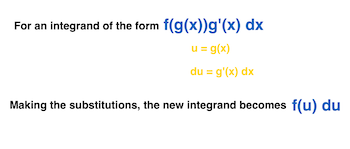#### Example 1: Calculate the integral {eq}\int_2^1 2xsin(x^2)dx {/eq}.

1) The integral is in the form {eq}\int_a^b f(g(x))g'(x) dx {/eq} where {eq}g(x) = x^2 {/eq} and g'(x) = 2x.

2) Setting u = g(x) gives {eq}u = x^2 {/eq}. Taking the derivative of u gives u' = 2xdx.

3) Making the appropriate substitutions into the integral {eq}\int_2^1 2xsin(x^2)dx {/eq} gives {eq}\int_2^1 sin(u)du {/eq}.

4) The boundaries of the integral become {eq}g(2) = 2^2 = 4 {/eq} and {eq}g(1) = 1^2 = 1 {/eq}.

5) Replacing the limits of integration with the new boundaries, {eq}\int_4^1 sin(u)du {/eq}, and evaluating the resulting integral gives:

{eq}- {/eq} the antiderivative of sin(x) is -cos(x) so the integral evaluates to {eq}-cos(u)|_4^1 {/eq}

{eq}- {/eq} evaluating the integral at the boundaries gives -cos(1) + cos(4) = -0.654.

#### Example 2: Evaluate the integral {eq}\int_{9.2}^{12.4} 6xln(3x^2) dx {/eq}.

1) The integral is in the form of {eq}\int_a^b f(g(x))g'(x) dx {/eq} where {eq}g(x) = 3x^2 {/eq} and g'(x) = 6x.

2) Setting u = g(x) gives {eq}u = 3x^2 {/eq}. Taking the derivative of u gives u' = 6xdx.

3) Making the appropriate substitutions into the integral {eq}\int_{9.2}^{12.4} 6xln(3x^2) dx {/eq} gives {eq}\int_{9.2}^{12.4} ln(u) du {/eq}.

4) The boundaries of the integral become {eq}g(9.2) = 3*9.2^2 = 253.92 {/eq} and {eq}g(12.4) = 3*12.4^2 = 461.28 {/eq}.

5) Replacing the limits of integration with the new boundaries, {eq}\int_{253.92}^{461.28} ln(u) du {/eq}, and evaluating the resulting integral gives:

{eq}- {/eq} the antiderivative of ln(u) is {eq}\frac{1}{u} {/eq} so the integral evaluates to {eq}\frac{1}{u}|_{253.92}^{461.28} {/eq}

{eq}- {/eq} evaluating the integral at the boundaries gives {eq}\frac{1}{461.28} - \frac{1}{253.92} = -0.002 {/eq}

#### Example 3: Evaluate the integral {eq}\int_{1}^{\pi} xe^{2x^2-1} dx {/eq}.

1) The integral is not in the form {eq}\int_a^b f(g(x))g'(x) dx {/eq}, and this example will show how to use the integrand to operate U-substitution. Here, {eq}g(x) = 2x^2-1 {/eq} and g'(x) = 4x.

2) Setting u = g(x) gives {eq}u = 2x^2-1 {/eq}. Taking the derivative of u gives du = 4xdx. This can be solved for dx so that {eq}\frac{du}{4x} = dx {/eq}.

3) Making the appropriate substitutions into the integral {eq}\int_{1}^{\pi} xe^{2x^2-1} dx {/eq} gives {eq}\int_{1}^{\pi} \frac{du}{4x}xe^{u} {/eq}. This is simplified to {eq}\frac{1}{4} \int_{1}^{\pi} e^{u} du {/eq}. Since all of the derivatives of u cancel out, there is an extra factor that can be pulled out of the integrand.

4) The boundaries of the integral become {eq}g(1) = 2*1^2-1 = 1 {/eq} and {eq}g(\pi) = 2* \pi^2-1 = 18.74 {/eq}.

5) Replacing the limits of integration with the new boundaries, {eq}\frac{1}{4} \int_{1}^{18.74} e^{u} du {/eq}, and evaluating the resulting integral gives:

{eq}- {/eq} the antiderivative of {eq}e^u {/eq} is {eq}e^u {/eq} so the integral evaluates to {eq}\frac{1}{4} e^u|_1^{18.74} {/eq}

{eq}- {/eq} evaluating the integral at the boundaries gives {eq}\frac{1}{4} (e^{18.74} - e^1) = 3.438*10^7 {/eq}

6) Alternatively:

{eq}- {/eq} {eq}\frac{1}{4} \int_{1}^{\pi} e^{u} du {/eq} evaluates to {eq}\frac{1}{4} e^{u} |_1^{\pi} {/eq}

{eq}- {/eq} replacing u with {eq}g(x) = 2x^2-1 {/eq} gives {eq}\frac{1}{4} e^{2x^2-1} |_1^{\pi} {/eq}

{eq}- {/eq} evaluating this gives {eq}\frac{1}{4} (e^{2*{\pi}^2-1} - e^{2*1^2-1}) = \frac{1}{4} (e^{18.74} - e^1) {/eq}

## U-Substitution to Find an Indefinite Integral

An indefinite integral is an integral without boundaries, and indefinite integrals are evaluated with an integration constant, C. During a u-substitution on an indefinite integral, since an indefinite integral has no boundaries, there is no need for changing limits of integration. To evaluate an indefinite integral using u-substitution, follow steps 1 - 3 of evaluating a definite integral using u-substitution. After step 3, solve the integral.

To unlock this lesson you must be a Study.com Member.

• Activities
• FAQs

## Changing the Limits of Definite Integrals: Practice Problems

In this activity, you'll assess your knowledge in using trigonometric substitution to solve definite integrals.

### Trigonometric Substitution

Here are the three cases on how to change variables via trigonometric substitution.

• Case 1: If the integrand has the form sqrt(a^2 - x^2) where a is greater than 0, then we introduce a variable theta by letting x = a sin(theta). This gives rise to sqrt(a^2 - x^2) = a cos(theta).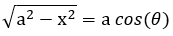• Case 2: If the integrand has the form sqrt(a^2 + x^2) where a is greater than 0, then we introduce a variable theta by letting x = a tan(theta). This gives rise to sqrt(a^2 + x^2) = a sec(theta).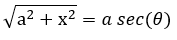• Case 3: If the integrand has the form sqrt(x^2 - a^2) where a is greater than 0, then we introduce a variable theta by letting x = a sec(theta). This gives rise to sqrt(x^2 - a^2) = a tan(theta).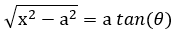• Blank paper
• Pencil

### Example

Before you answer the practice problems, let us first look at the steps in evaluating the expression below: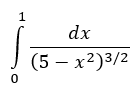Step 1: Determine the case where the integrand sqrt(sqrt(5)^2 - x^2) belongs. By inspection, one can see that the integrand follows case 1, where a = sqrt(5).

Step 2: Now, let x = sqrt(5)sin(theta) and solve for dx in terms of d(theta).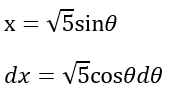Step 3: It follows from case 1 that the integrand will be equal to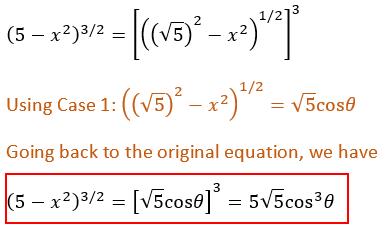Step 4: Rewrite and evaluate the integral using the equations obtained in Step 2 and 3.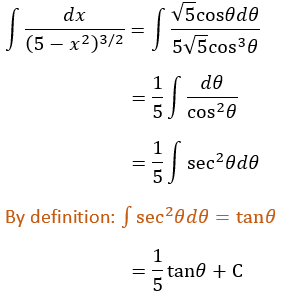Step 5: Change the limits by using the equation in Step 2,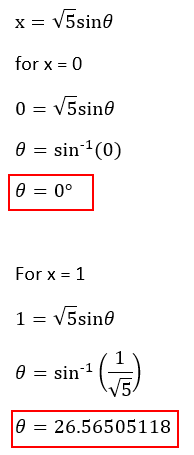Step 6: Evaluate Step 4 using the new limits.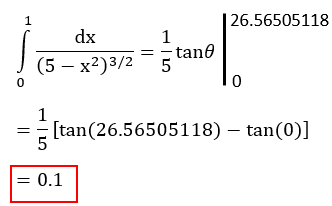Therefore, evaluating the integral via trigonometric substitution yields 0.1.

### Practice Problems

Now let's see if you understand the steps outlined above. With your pen and paper, provide clear solutions and answers to the given problems.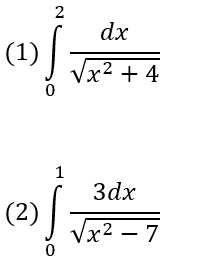#### How to find the upper and lower bounds of an integral?

To find the upper and lower boundaries of the new integral in the U-substitution, identify which function is g(x). Evaluate g(x) at each boundary, and the results are the new lower and upper boundaries.

#### How to change variables in integration?

To change integration variables, first identify one function, g(x), in the integrand to be 'u'.Take the derivative of this function, du = g'(x)dx. Replace g(x) with u and g'(x)dx with du. Finally, integrate over u.

#### What is U substitution in calculus?

In calculus, U-substitution is a method to calculate integrals whose integrands contain composite functions. U-substitution is the integral version of the derivative chain rule.

#### Can U substitution be used for definite integrals?

Yes. U-substitution can solve definite integrals as long as an appropriate change of boundaries is made. U-substitution can also solve indefinite integrals with no change of limits.

### Register to view this lesson

Are you a student or a teacher?